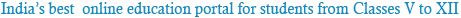### NCERT Solutions for Class 6th Science: Chapter 10: Motion and Measurement of Distances

Question 1:
Give two examples each of modes of transport used on land, water, and air.

Two examples of modes of transport used on land are buses and wheel carts.
Two examples of modes of transport used on water are ships and boats.
Two examples of modes of transport used in air are aeroplanes and helicopters.

Question 2:
Fill in the blanks:
(i) One metre is ______________ cm.
(ii) Five kilometres is ______________ m.
(iii) Motion of a child on a swing is ______________.
(iv) Motion of the needle of a sewing machine is ______________.
(v) Motion of wheel of a bicycle is______________.

(i) One metre is 100 cm.
(ii) Five kilometres is 5000 m.
1 km = 1000 m
5 km = 1000 × 5 = 5000 m
Hence, the answer is 5000 m.
(iii) Motion of a child on a swing is periodic.

Periodic motion:
The motion of a swing repeats itself at a certain time interval. Therefore, it has periodic motion. Hence, a child on a swing is said to have periodic motion.
(iv) Motion of the needle of a sewing machine is periodic.
Periodic motion:
The needle of a sewing machine moves up and down repeatedly with a certain time interval. Hence, it is an example of periodic motion.
(v) Motion of the wheel of a bicycle is circular.
Circular motion:
The central part of the wheel of a bicycle is attached to a fixed point. The wheel rotates about this fixed point as the bicycle moves. Hence, the wheel has circular motion.

Question 3:
Why can a pace or a footstep not be used as a standard unit of length?

The size of the foot varies from person to person. If footsteps of two persons are used to measure the length respectively, then the two distances may not be equal. Thus, a footstep is not a constant quantity. Hence, it cannot be used as a standard unit of length.

Question 4:
Arrange the following lengths in their increasing magnitude:
1 metre, 1 centimetre, 1 kilometre, 1 millimetre
1 cm = 10 mm
1 m = 100 cm = 1000 mm
Again, 1 km = 1000 m = 100000 cm = 10000000 mm.
Hence, 1 mm is smaller than 1 cm, 1 cm is smaller than 1 m, and 1 m is smaller than 1 km, i.e.,Question 5:
The height of a person is 1.65 m. Express this in cm and mm.

Height of the person = 1.65 m
1 m = 100 cm
1.65 m = 100 × 1.65 = 165 cm
Hence, the height of the person is 165 cm.
Again, 1 m = 100 cm = 1000 mm
Therefore, 1.65 m = 1.65 × 10 = 1650 mm
Hence, the height of the person is 1650 mm.

Question 6:
The distance between Radha’s home and her school is 3250 m. Express this distance in km.

The distance between Radha’s home and her school is 3250 m.
1 km = 1000 m
i.e., 1000 m = 1 kmQuestion 7:
List the common characteristics of living things.

Some common characteristics of living things are that they
(a) require food
(b) respire and excrete waste material
(c) respond to stimuli in their environment
(d) reproduce to maintain their number
(e) move from one place to another
(f) grow and die

Question 8:
Write the similarities and the differences between the motion of a bicycle and a ceiling fan that has been switched on.

Similarities between the motion of a bicycle and a ceiling fan:
(i) The blades of a fan and the wheels of a bicycle are fixed at a point.
(ii) Both have circular motion about their respective fixed points.
Differences between the motion of a bicycle and a ceiling fan:
(i) A bicycle has linear motion, whereas the blades of a ceiling fan do not have linear motion.
(ii) The motion of the blades of a fan is periodic, whereas the motion of a bicycle is rectilinear motion.

Question 9:
Why can you not use an elastic measuring tape to measure distance? What would be some of the problems you would meet in telling someone about a distance you measured with an elastic tape?

An elastic measuring tape is stretchable. It cannot be used to measure distances because the length of the tape may change on stretching. As a result, the measured length would not be correct.
If you measure the length of an object twice using an elastic tape, then you may get different values of the same length each time. This is because elastic tapes are stretchable.

Question 10:
Give two examples of periodic motion.

Examples of periodic motion:
(i) Motion of a pendulum
The bob of a pendulum repeats itself at a certain time period. This motion is called periodic motion.
(ii) Motion of a boy sitting on a swing
The motion of a swing repeats itself at a certain time period. Hence, a boy sitting on a swing has periodic motion.Powered by: iVerticals Web Technology Pvt Ltd